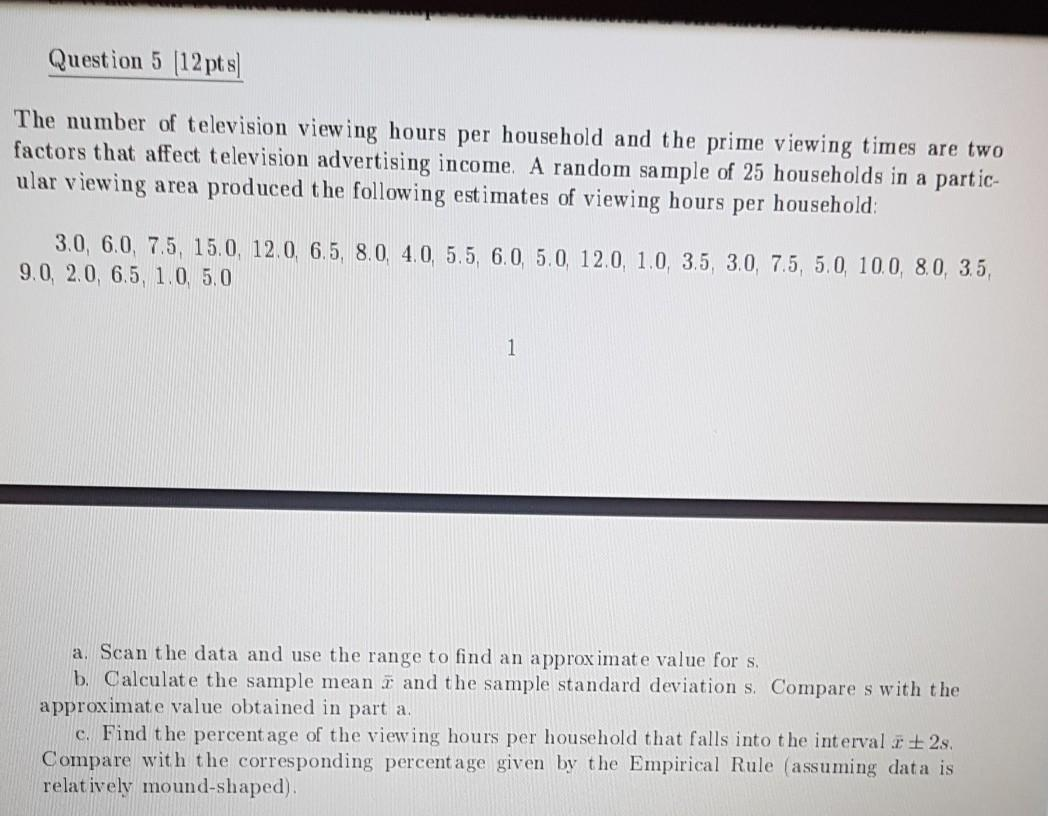# Question Solved1 AnswerQuestion 5 (12 pts) The number of television viewing hours per household and the prime viewing times are two factors that affect television advertising income. A random sample of 25 households in a partic- ular viewing area produced the following estimates of viewing hours per household 3.0, 6.0, 7.5, 15.0, 12.0, 6.5, 8.0, 4.0, 5.5, 6.0 5.0 12.0, 1.0, 3.5, 3.0, 7.5, 5.0, 100, 8.0, 3.5, 9.0, 2.0, 6.5, 1.0, 5.0 1 a. Scan the data and use the range to find an approximate value for s. b. Calculate the sample mean i and the sample standard deviation s. Compare s with the approximate value obtained in part a, c. Find the percentage of the viewing hours per household that falls into the interval : +2s. Compare with the corresponding percentage given by the Empirical Rule (assuming data is relatively mound-shaped).THRTFR The Asker · Probability and StatisticsTranscribed Image Text: Question 5 (12 pts) The number of television viewing hours per household and the prime viewing times are two factors that affect television advertising income. A random sample of 25 households in a partic- ular viewing area produced the following estimates of viewing hours per household 3.0, 6.0, 7.5, 15.0, 12.0, 6.5, 8.0, 4.0, 5.5, 6.0 5.0 12.0, 1.0, 3.5, 3.0, 7.5, 5.0, 100, 8.0, 3.5, 9.0, 2.0, 6.5, 1.0, 5.0 1 a. Scan the data and use the range to find an approximate value for s. b. Calculate the sample mean i and the sample standard deviation s. Compare s with the approximate value obtained in part a, c. Find the percentage of the viewing hours per household that falls into the interval : +2s. Compare with the corresponding percentage given by the Empirical Rule (assuming data is relatively mound-shaped).
More
Transcribed Image Text: Question 5 (12 pts) The number of television viewing hours per household and the prime viewing times are two factors that affect television advertising income. A random sample of 25 households in a partic- ular viewing area produced the following estimates of viewing hours per household 3.0, 6.0, 7.5, 15.0, 12.0, 6.5, 8.0, 4.0, 5.5, 6.0 5.0 12.0, 1.0, 3.5, 3.0, 7.5, 5.0, 100, 8.0, 3.5, 9.0, 2.0, 6.5, 1.0, 5.0 1 a. Scan the data and use the range to find an approximate value for s. b. Calculate the sample mean i and the sample standard deviation s. Compare s with the approximate value obtained in part a, c. Find the percentage of the viewing hours per household that falls into the interval : +2s. Compare with the corresponding percentage given by the Empirical Rule (assuming data is relatively mound-shaped).